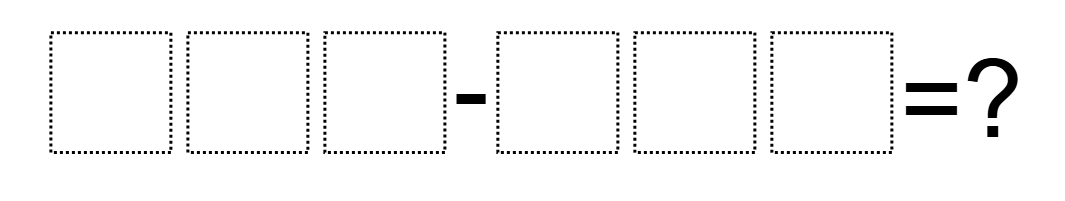Home > Grade 2 > Subtraction to Get the Smallest Difference

# Subtraction to Get the Smallest Difference

Directions: Using the digits 1 to 9, at most one time each, fill the boxes below to create the smallest possible difference.### Hint

Remember that zeros are not allowed.  Because you can only use each digit once, the digit in the hundreds place can’t be the same.

14

One possible solution is 612-598 = 14.

Source: Graham Fletcher

## Time Twister

Directions: Using the digits 0 to 9, at most one time each, create three different …

1.Cameron Williams (Mrs. Elliott's 4th Graders)

We also got 14 with 412-398=14.

•Nice!

•999-987

2.But you used 1 in the question and 1 in the answer.

•The answer isn’t included in the digits being used once, since it says “fill the boxes”.

3.My best is 718-695 = 23

4.Jocelyn Rodriguez

612-598=14.

5.612-598=14

6.612-598 = 14

7.612-598=14

8.Sydney Molitoris

612-598=14

9.612-598=14

10.412-398=14

11.512-498=14

12.512-498=14

13.My best is 701-698=3

•You cannot use the number 0

•516-498=18

14.You cannot use the number 0 in the problem.

15.612-598=14

16.516-498=18

17.18.612-598=14

19.987-987=0

•you can’t repeat the same numbers

20.102-96=2